#Sign of a Quadratic Function with Application to InequalitiesMany inequalities lead to finding the sign of a quadratic expression. let us discuss this problem here. Consider the quadratic function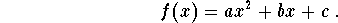We know that

1
if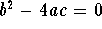(double root case), then we have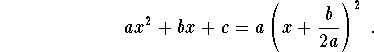In this case, the function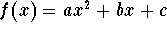has the sign of the coefficient a.

 a<0 a>0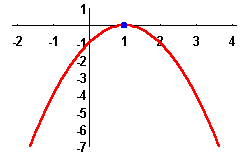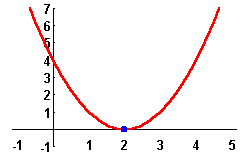2
If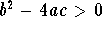(two distinct real roots case). In this case, we have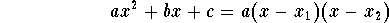where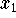and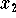are the two roots with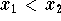. Since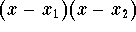is always positive when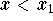and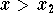, and always negative when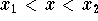, we get

•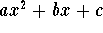has same sign as the coefficient a whenand;
•has opposite sign as the coefficient a when.
 a<0 a>0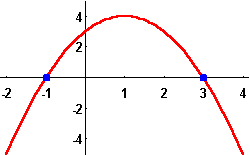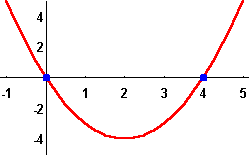3
If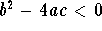(complex roots case), thenhas a constant sign same as the coefficient a.

 a<0 a>0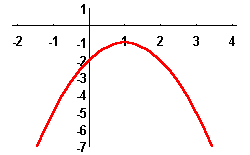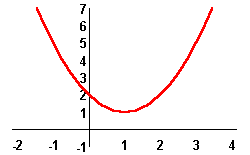Example: Solve the inequality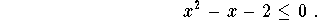Solution. First let us find the root of the quadratic equation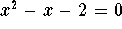. The quadratic formula gives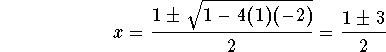which yields x= -1 or x=2. Therefore, the expression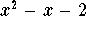is negative or equal to 0 when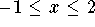.[Algebra] [Complex Variables]
[Geometry] [Trigonometry ]
[Calculus] [Differential Equations] [Matrix Algebra]S.O.S MATHematics home page

Do you need more help? Please post your question on our S.O.S. Mathematics CyberBoard.Author: Mohamed Amine Khamsi